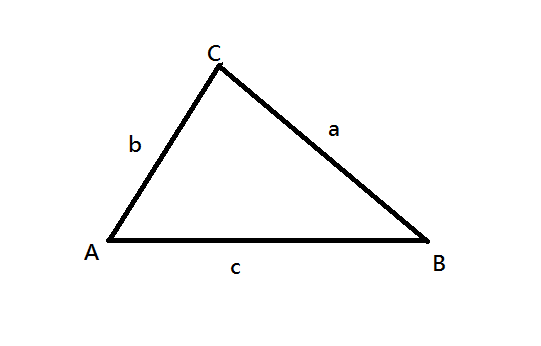# Maximum Perimeter Triangle with Greedy ApproachLet’s think of an array of stick-lengths, find which three sticks form a non-degenerate triangle such that: the triangle has a maximum perimeter if there are two or more combinations with the same value of maximum perimeter, output the one with the longest side. Output -1 if not possible

Let’s think of an array of stick-lengths, find which three sticks form a non-degenerate triangle such that:

• the triangle has a maximum perimeter
• if there are two or more combinations with the same value of maximum perimeter, output the one with the longest side.
• Output -1 if not possible

Are you confusing about the non-degenerate triangle?

If a, b and c are the sides of the triangle, and if the following 3 conditions are true, then it is a non-degenerate triangle.non-degenerate triangle

``````a + b > c
a + c > b
b + c > a``````

For example, assume there are stick lengths _**_sticks = [ 1,3,5,10,4,2]_. The triplet _(1,2,3)_ will not form a triangle. Neither _(4,5,6) __will or _(2,3,5)**, so the problem is reduced to **(2,3,4) _and _(3,4,5)**. The longer perimeter is **(3,4,5) =12_**_ ._

So many conditions, but this is really easy to solve.

From all the possible triplets, check for the given conditions and keep track of the maximum ones. In the end, output the triplet with the longest side. Yes. Correct. But there will be a problem…

## How to Install OpenJDK 11 on CentOS 8

What is OpenJDK? OpenJDk or Open Java Development Kit is a free, open-source framework of the Java Platform, Standard Edition (or Java SE).

## A greedy algorithm is a simple

The Greedy Method is an approach for solving certain types of optimization problems. The greedy algorithm chooses the optimum result.

## Java Core (2020) | Java for beginners | Brush up your Java Skills | Get Hired

In this video we will learn about all the major concepts that come under Java Core . The course is very carefully designed and is made with keeping simplicit...

## Getting Started with dynamic programming

Dynamic Programming is mainly an optimization over plain recursion. Wherever we see a recursive solution that has repeated calls for the same inputs.

## MO’s Algorithm — Range Queries Made Easy

An Efficient Way to Solve Offline Range Query Problems. MO’s Algorithm aka Square Root Decomposition, a very efficient and easy technique to solve Range Query Problems (RQP).